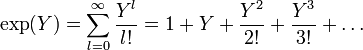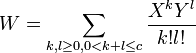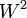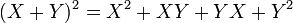# Deducing the Baker-Campbell-Hausdorff formula from associative algebra manipulation

(diff) ← Older revision | Latest revision (diff) | Newer revision → (diff)

## Statement

### Infinite version

The Baker-Campbell-Hausdorff formula in the simply connected case (and hence also the nilpotent case, at least in large characteristic) can be computed by the following procedure.

• Start with the power series for the exponential function$\exp(X)$ and$\exp(Y)$ viewed as elements of the formal power series ring$\mathbb{Q}\langle X,Y \rangle$ with$X,Y$ non-commuting variables:$\! \exp(X) = \sum_{k=0}^\infty \frac{X^k}{k!} = 1 + X + \frac{X^2}{2!} + \frac{X^3}{3!} + \dots$$\! \exp(Y) = \sum_{l=0}^\infty \frac{Y^l}{l!} = 1 + Y + \frac{Y^2}{2!} + \frac{Y^3}{3!} + \dots$

Now, multiply both of these:$\! \exp(X)\exp(Y) = \sum_{k=0}^\infty \sum_{l=0}^\infty \frac{X^kY^l}{k!l!}$

Subtract 1 and define$W = \exp(X)\exp(Y) - 1$. We have:$\! W = \exp(X) + \exp(Y) + \sum_{k=1}^\infty \sum_{l=1}^\infty \frac{X^kY^l}{k!l!}$

The Baker-Campbell-Hausdorff formula is obtained by applying the power series for$\log(1 + W)$ and plugging the above expression for$W$ into that. Ultimately, we have to convert terms that are obtained in terms of$X,Y$ into commutators and iterated commutators.

### Truncated version for nilpotent groups

In the case of a group of nilpotency class at most$c$, we can simplify the computations above as follows: we can work within a ring where all products of length$c + 1$ or more are zero. Thus, in here, we can assume that:$\! \exp(X) = \sum_{k=0}^{c-1} \frac{X^k}{k!} = 1 + X + \frac{X^2}{2!} + \frac{X^3}{3!} + \dots + \frac{X^{c-1}}{(c - 1)!}$$\! \exp(Y) = \sum_{l=0}^{c-1} \frac{Y^l}{l!} = 1 + Y + \frac{Y^2}{2!} + \frac{Y^3}{3!} + \dots + \frac{Y^{c-1}}{(c - 1)!}$

Now, multiply both of these and remove all terms where the total exponent is$c + 1$ or more:$\! \exp(X)\exp(Y) = \sum_{k,l \ge 0, k + l \le c} \frac{X^kY^l}{k!l!}$

Subtract 1 and define$W = \exp(X)\exp(Y) - 1$. We have:$\! W = \sum_{k,l \ge 0, 0 < k + l \le c} \frac{X^kY^l}{k!l!}$

Now apply the power series for$\log(1 + W)$. Note that this power series again can be terminated after$c$ terms.

## Particular cases

We consider the cases for various nilpotent groups for fixed bounds on nilpotency class.

### Case of abelian group

In this case, we work with non-commuting variables$X,Y$ such that$X^2 = XY = YX = Y^2 = 0$. Thus:$\exp(X) = 1 + X$$\exp(Y) = 1 + Y$

We thus get:$\exp(X)\exp(Y) = (1 + X)(1 + Y) = 1 + X + Y + XY = 1 + X + Y$

(we drop the$XY$ term because it equals zero).

Thus:$W = X + Y$

We thus get:$\log(1 + W) = W - \frac{W^2}{2} + \frac{W^3}{3} - \dots = W = X + Y$

Thus, the Baker-Campbell-Haudorff formula gives$X + Y$.

Higher order terms become zero because of all terms involving products of length two or more are zero.

### Case of class two

In this case, we work with non-commuting variables$X,Y$ such that$X^3 = X^2Y = XYX = XY^2 = YX^2 = YXY = Y^2X = Y^3 = 0$. Thus:$\! \exp(X) = 1 + X + \frac{X^2}{2}$$\! \exp(Y) = 1 + Y + \frac{Y^2}{2}$

We thus get:$\exp(X)\exp(Y) = \left(1 + X + \frac{X^2}{2}\right)\left(1 + Y + \frac{Y^2}{2}\right) = 1 + X + \frac{X^2}{2} + Y + XY + \frac{X^2Y}{2} + \frac{Y^2}{2} + \frac{XY^2}{2} + \frac{X^2Y^2}{4}$

We drop all products of degree three or more and rearrange the remaining terms to get:$\! \exp(X)\exp(Y) = 1 + X + Y + \frac{X^2}{2} + XY + \frac{Y^2}{2}$

We thus get:$\! W = \exp(X)\exp(Y) - 1 = X + Y + \frac{X^2}{2} + XY + \frac{Y^2}{2}$

Finally, we compute$\log(1 + W)$. We have:$\log(1 + W) = W - \frac{W^2}{2}$

We note that$W^2$ is the same as the square of its linear part because the square of the degree two part, as well as products of the degree two and the linear part, are degree three or more and hence zero. Thus:$\log(1 + W) = X + Y + \frac{X^2}{2} + XY + \frac{Y^2}{2} - (X + Y)^2/2$

Simplifying, we get:$\log(1 + W) = X + Y + \frac{X^2 + 2XY + Y^2 - (X + Y)^2}{2}$

Now note that:$\! (X + Y)^2 = X^2 + XY + YX + Y^2$

Plugging this in, we get:$\log(1 + W) = X + Y + \frac{X^2 + 2XY + Y^2 - (X^2 + XY + YX + Y^2)}{2}$

Simplifying, we get:$\log(1 + W) = X + Y + \frac{XY - YX}{2}$

Rewrite$XY - YX = [X,Y]$ and we get the formula:$\! X + Y + \frac{1}{2}[X,Y]$